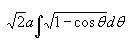# Thread: An integration of sorts.

1. ## An integration of sorts.That's the question I can't do. It would be so much easier if it was a cos squared!

I think I have to use a substitution but i'm not quite sure what it should be.2.Originally Posted by Showcase_22That's the question I can't do. It would be so much easier if it was a cos squared!

I think I have to use a substitution but i'm not quite sure what it should be.Multiply by $\displaystyle \frac{\sqrt{1+\cos{\theta}}}{\sqrt{1+\cos{\theta}} }$

Can you do it now?

3. Or, you can rewrite $\displaystyle \sqrt{1-\cos{\theta}}$ as $\displaystyle \sqrt{2}\sin{\left(\frac{x}{2}\right)}$. I'll leave it to you to figure out why they're equivalent.

4. I can do it, and I have solved it.I tried deriving the second identity myself but I couldn't figure that part out.

can you show me how it's done?

5. $\displaystyle \sqrt{1-\cos{\theta}}$

$\displaystyle = \displaystyle\sqrt{\underbrace{1-\cos^2{\frac{\theta}{2}}}_{\sin^2{\frac{\theta}{2} }}+\sin^2{\frac{\theta}{2}}}$

$\displaystyle = \sqrt{2\sin^2{\frac{\theta}{2}}}$

$\displaystyle = \sqrt{2}\sin{\frac{\theta}{2}}$

6. oh!! I don't know what I was doing. It's just a simple double angle formulae.

That's rather useful.#### Search Tags

integration, sorts